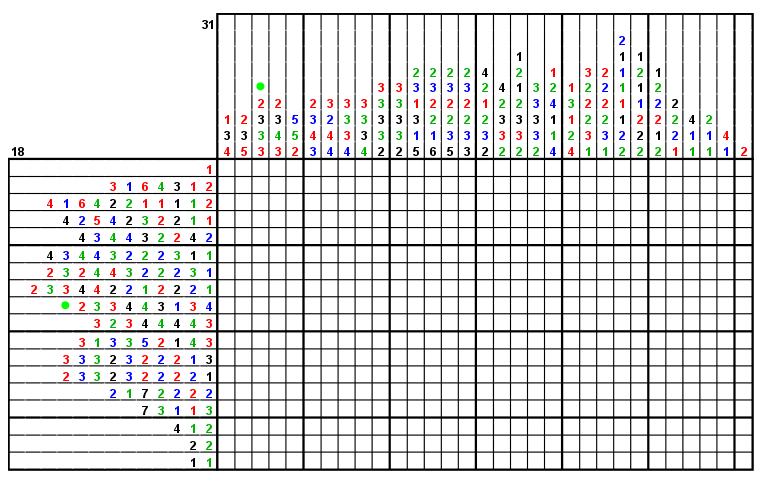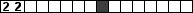# Introduction

Naoyuki Tamura has described a Nonogram Solver written in Copris, a Constraint Programming DSL embedded in Scala. When I tested it for my Survey of Paint-by-Number Puzzle Solvers, I was impressed by it's ability to solve many hard puzzles that previous solvers I had tested had not been able to solve. I was also impressed by the simplicity of the Nonogram solving program, which does little more than parse the input, post the constraints, and pretty print the solution.

Nonogram solving has become a standard test problem for designers of constraint solving systems. Typically only black-and-white nonograms are considered. I thought it might be interesting to see how the approach extends to a slightly more complex problem: multicolor nonograms.

An example of a multicolor nonogram is shown below:Here the numeric clues describe a five-color picture, with black, red, green and blue cells on a white background. As in a standard nonogram, the clues describe the sizes of each block of cells, but now the color of the number tells the color of the block of cells. So we know the first column of the puzzle contains a single red cell, followed by a block of three consecutive black cells, followed by a block of four consecutive red cells. The exact position of those three blocks in the column is what the solver must determine. Note that two blocks must be separated by at least one white cell if they are of the same color, but there need be no space between them if they are of different colors.

If you'd like to try solving the puzzle above, you can do so on webpbn.com.

# Constraint Model

Extending Tamura's constraint model to multicolor puzzles is pretty straight forward.

## Input

We'll need a little more input. For each clue number we need to know not only the value but the color. For simplicity, we are simply going to represent each color by a number. Zero is always the background color, so no clues will have that color. The other colors are just 1,2,3 and so on.

Black & White Multicolor
 m : number of rows n : number of columns rows(i)(k) : run length of the k-th block in the i-th row (i=0 .. m-1) cols(j)(k) : run length of the k-th block in the j-th column (j=0 .. n-1)
 m : number of rows n : number of columns nc : number of colors (not including background color) rown(i)(k) : run length of the k-th block in the i-th row (i=0 .. m-1) rowc(i)(k) : color of the k-th block in the i-th row (i=0 .. m-1) coln(j)(k) : run length of the k-th block in the j-th column (j=0 .. n-1) colc(j)(k) : color of the k-th block in the j-th column (j=0 .. n-1)

## CSP Variables

The only change to the CSP variables is that is that the color of a cell can now have more values.

 Black & White Multicolor Color of cell (i,j): x(i,j) ∈ { 0,1 } Left-most position of the k-th block in the i-th row: r(i,k) ∈ { 0..n - rows(i)(k) } Top-most position of the k-th block in the j-th column: c(i,j) ∈ { 0..n - cols(j)(k) } Color of cell (i,j): x(i,j) ∈ { 0..nc } Left-most position of the k-th block in the i-th row: r(i,k) ∈ { 0..n - rown(i)(k) } Top-most position of the k-th block in the j-th column: c(i,j) ∈ { 0..n - coln(j)(k) }

## Constraints

The constraints, of course, also need to be slightly modified.

The non-overlapping constraints are the same for pairs of blocks of the same color, but if they are different colors the '<' sign becomes a '≤' because there does not need to be a separating white space.

The covering constraints for black and white puzzles said that a cell could be black if and only if it was covered by a block. We say instead that a cell can be a given color if and only if it is covered by a block of that same color.

 Black & White Multicolor Row blocks do not overlap: r(i,k)+rows(i)(k) < r(i,k+1) Column blocks do not overlap: c(j,k)+cols(j)(k) < c(j,k+1) Row blocks do not overlap: When rowc(i)(k) = rowc(i)(k+1): r(i,k)+rown(i)(k) < r(i,k+1) When rowc(i)(k) ≠ rowc(i)(k+1): r(i,k)+rown(i)(k) ≤ r(i,k+1) Column blocks do not overlap: When colc(i)(k) = colc(i)(k+1): c(j,k)+coln(j)(k) < c(j,k+1) When colc(i)(k) ≠ colc(i)(k+1): c(j,k)+coln(j)(k) ≤ c(j,k+1) The cell (i,j) is black iff it is contained in a row block: x(i,j)=1 ↔ ∨k (r(i,k) ≤ j ∧ j < r(i,k)+rows(i,k)) The cell (i,j) is black iff it is contained in a column block: x(i,j)=1 ↔ ∨k (c(j,k) ≤ i ∧ i < c(j,k)+cols(j,k)) The cell (i,j) is color v iff it is contained in a row block of that color: x(i,j)=v ↔ ∨k : rowc(i,k)=v (r(i,k) ≤ j ∧ j < r(i,k)+rown(i,k)) The cell (i,j) is black iff it is contained in a column block: x(i,j)=v ↔ ∨k : colc(j,k)=v (c(j,k) ≤ i ∧ i < c(j,k)+coln(j,k))

This set of constraints may appear to be much more complex than the original one, but they really aren't. We have the same number of non-overlapping constraints, but now some contain '≤' signs instead of '<' signs. Originally we had two covering constraints for each grid cell, one for rows and one for columns. Now there are two constraints for each non-background color, but the constraints for color v contain only the disjunctive terms corresponding to the clues of that color, so the total "length" of the constraints is about the same.

It's also important to note that in the special case where the number of non-background colors is one, the multicolor case is identical to the black and white case. That means the multicolor solver shouldn't be significantly slower on black and white puzzles than the original version was.

## Implementation

Modifying Tamura's program for this generalized formulation was not difficult. I'd never heard of the Scala language before, and only briefly skimmed some slightly murky online documentation. I read no Copris documentation at all. Mostly I just followed the patterns of Tamura's code. Very likely I have implemented some things less gracefully than they would be implemented by someone who actually knew the language.

Debugging proved mildly difficult, since Scala is compiled into Java byte code in such a way that at run time it is unable to report what line of Scala code caused an error. This is obnoxious and stupid, but inserting a lot of print statements into the program made it easy enough to localize the errors. Overall, writing and debugging the program took a couple hours - less time than writing this report.

The complete Scala program is available here.

To compile and run it, you'll need to download the source code zip file from Tamura's page and simply substitute my Scala script for his.

Of course, the input files for the new program are in a different format than the ones for the black and white program, since they need to include clue color data. They are CWC files instead of CWD files, and can be exported from my Webpbn Puzzle Export Page.

When I modified the subroutine that prints out the solution of the puzzle, I did it in a slightly dimwitted way that will not work well for puzzles with more than nine non-background colors, but since webpbn supports only four non-background colors, that will suffice for now. Doing it better would have required learning more Scala.

## Conclusions

I'm not sure if I would recommend that multicolor nonograms replace black and white nonograms as a standard test problem for solvers. Obviously multicolor nonograms can be just as hard as black and white ones, but in practice they tend to be easier on average. The first row shown below has many more possible solutions than the second:So this generalized version of the problem doesn't really tend to be a more stringent test of the solver's capabilities.

The color nonogram solver that resulted from this exercise is a lot slower on most puzzles than the pbnsolve program that I wrote from scratch in C, but initial tests indicate that, like Tamura's original black and white puzzle solver, it can solve many more of the rare hard puzzles. In fact, it was able to solve every one of the 13,456 multicolor puzzles I tested it on, though one took over two hours to solve.

The pbnsolve program solves almost as many puzzles, and does the vast majority much faster, but it is the product of years of work and refinement. The Copris solver took me only a few hours to build. Pbnsolve consists of some 9,000 lines of application-specific code, compared to some 120 lines for this program.

I am only aware of about four programs that solve color nonograms, and only two of them are any good at solving hard puzzles. On the other hand, there are dozens of black and white nonogram solvers. If you wanted to extend one of those to work for color puzzles, you'd find yourself rewriting most of it. Data structures would have to change. The line solver algorithm used for multicolor puzzles is a great deal more complex than for black and white puzzles. Search heuristics would have to change.

With Copris, on the other hand, I was able to make the upgrade quite easily, in spite of the fact that I know very little about Scala, less about Copris, and have never previously done any programming with any Constraint Programming System.

Frankly, I think this is gigantic. The ability afforded by modern CSPs to easily build powerful solvers for complex problems is truly amazing, and I strongly suspect that this is a very important part of the future of computer science.